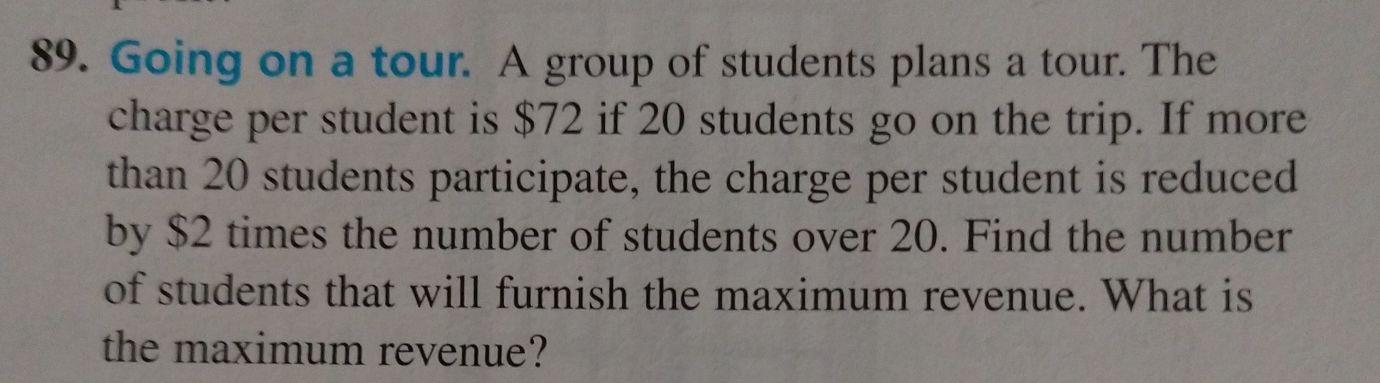### ¿Todavía tienes preguntas de matemáticas?

Pregunte a nuestros tutores expertos
Algebra
Pregunta89. Going on a tour. A group of students plans a tour. The charge per student is $$\ 72$$ if $$20$$ students go on the trip. If more than $$20$$ students participate, the charge per student is reduced by $$\ 2$$ times the number of students over $$20 .$$ Find the number of students that will furnish the maximum revenue. What is the maximum revenue?

$$f(x) = (20+ x)(72- 2x) = 1440+ 32x- 2x^2$$ ,
$$f'(x) = 32- 4x = 0 , x = 8$$ , hence 28 students , revenue = \$1568